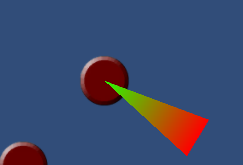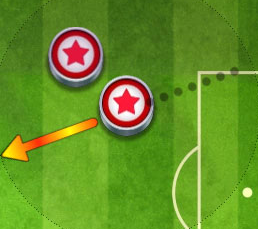# Making a arrow instead of Linerenderer

Hi all,

I’m trying to create something like an 2D Curling Game, so far i have the curling physics almost down but i’m having difficulties in figuring out how to draw a nice indicator of the force used to hit the curling (MouseDrag).

Currently i have a LineRenderer that is drawn on MouseDrag event, it looks like this:It looks ok but i instead of this LineRenderer i would like to have an arrow drawn in the size of the drag size or the force used to push the curling ball as well as the direction, something similar to the arrows drawn in a game called soccer stars, something like this:Can please somebody help me figure this out, here is also the code i have written so far:

``````using UnityEngine;
using System.Collections;

public class Controller : MonoBehaviour
{
public float start_x, start_y, end_x, end_y, force_x, force_y;
public int force = 60;
//public GameObject arrow;

//Taking the initial position of the mouse
void OnMouseDown()
{
start_x = GameObject.Find("Puck").transform.position.x;
start_y = GameObject.Find("Puck").transform.position.y;

//arrow = Instantiate(Resources.Load("Arrow"),new Vector3(start_x,start_y,1f),Quaternion.identity) as GameObject;

}

//Taking the end position of the mouse and drawing a line renderer
void OnMouseDrag()
{
GameObject.Find("Line").transform.position = Camera.main.ScreenToWorldPoint(Input.mousePosition);
GameObject.Find("Line").transform.position = new Vector3(GameObject.Find("Line").transform.position.x, GameObject.Find("Line").transform.position.y, 0);

end_x = GameObject.Find("Line").transform.position.x;
end_y = GameObject.Find("Line").transform.position.y;

//arrow.transform.position = Camera.main.ScreenToWorldPoint(Input.mousePosition);
//arrow.transform.position = new Vector3(GameObject.Find("Line").transform.position.x, GameObject.Find("Line").transform.position.y, 0);
//arrow.transform.rotation = GameObject.Find("Line").transform.rotation;

if(GetComponent<LineRenderer>() != null)
{
GetComponent<LineRenderer>().enabled = true;
GetComponent<LineRenderer>().SetVertexCount(2);
GetComponent<LineRenderer>().SetPosition(0, new Vector3(transform.position.x, transform.position.y, 1));
GetComponent<LineRenderer>().SetPosition(1, new Vector3(GameObject.Find("Line").transform.position.x, GameObject.Find("Line").transform.position.y, 1));
GetComponent<LineRenderer>().SetWidth(0f, 1f);
GetComponent<LineRenderer>().sortingLayerName = ("myLayer");
GetComponent<LineRenderer>().sortingOrder = 10;
}
}

//Calculating the force
void OnMouseUp()
{
force_x = start_x - end_x;
force_y = start_y - end_y;

//Hide LineRenderer if one is attached
if(GetComponent<LineRenderer>() != null)
{
GetComponent<LineRenderer>().enabled = false;
}

//Destroy(arrow);

//Turning on gravity if needed
//GetComponent<Rigidbody2D>().gravityScale = 1;
}

void Update()
{
GetComponent<Rigidbody2D>().drag = 0.9f;
}

}
``````

Thank you in forward.

With Unity3D 5.5 you can use the WidthCurve of the LineRenderer to make arrows. Be careful to place the line positions to match that width curve. Otherwise the mesh won’t be generated correctly. Here’s a script:

``````using UnityEngine;

[RequireComponent(typeof(LineRenderer))]
public class LineRendererArrow : MonoBehaviour
{
[Tooltip("The percent of the line that is consumed by the arrowhead")]
[Range(0, 1)]
public Vector3 ArrowOrigin;
public Vector3 ArrowTarget;
private LineRenderer cachedLineRenderer;

void Start()
{
UpdateArrow();
}

private void OnValidate()
{
UpdateArrow();
}

void UpdateArrow()
{
if (cachedLineRenderer == null)
cachedLineRenderer = this.GetComponent<LineRenderer>();

cachedLineRenderer.widthCurve = new AnimationCurve(
new Keyframe(0, 0.4f)
, new Keyframe(0.999f - PercentHead, 0.4f)  // neck of arrow
, new Keyframe(1 - PercentHead, 1f)  // max width of arrow head
, new Keyframe(1, 0f));  // tip of arrow
cachedLineRenderer.SetPositions(new Vector3[] {
ArrowOrigin
, Vector3.Lerp(ArrowOrigin, ArrowTarget, 0.999f - PercentHead)
, Vector3.Lerp(ArrowOrigin, ArrowTarget, 1 - PercentHead)
, ArrowTarget });
}
}
``````

You will need to either find or create an arrow sprite of your own. Then position,scale and rotate it according to your input and controls. There is no built in unity way to draw an arrow other than the Handles library but this is used for custom editors and not game time drawing.